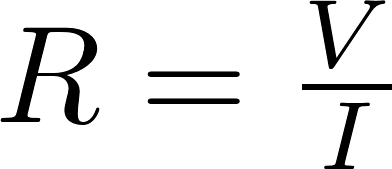# Resistance

The resistance is a measure of the opposition of to the flow of current of an object. The symbol for resistance is R and it is measured in ohm (Ω). The resistance is different for each material. Some materials like rubber have a high resistance and others like copper have a very low resistance.

### Ohm's law

Ohm's law

Ohm's law describes the relation between Voltage, Current and Resistance. The law states that the voltage is equal to the current multiplied by the resistance. Ohm's law is named after the German physicist Georg Ohm.

### Formula

The resistance can be calculated by dividing the voltage by the current:R is the symbol for resistance and is measured in ohm (Ω).
V is the symbol for voltage and is measured in volt (V).
I is the symbol for current and is measured in ampere (A).

### Resistivity

Resistivity is the property of a metal to resist the flow of current. The resistivity is different for each material and it is measured in ohm-meter (Ω⋅m).

material resistivity ρ 10⁻⁹Ω⋅m
silver 15.9
copper 16.8
aluminium 26.5
tungsten 56
iron 97.1
platinum 106
manganin 482